# 对非均匀采样信号进行重采样

### 以所需采样率对非均匀采样信号进行重采样

`resample` 函数允许您将非均匀采样信号转换为使用新的均匀采样率。

```rng default nominalFs = 48000; f = 500; Tx = 0:1/nominalFs:0.01; irregTx = sort(Tx + 1e-4*rand(size(Tx))); x = sin(2*pi*f*irregTx); plot(irregTx,x,'.')```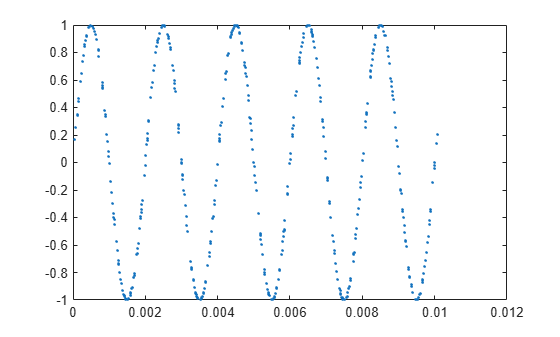```desiredFs = 44100; [y, Ty] = resample(x,irregTx,desiredFs); plot(irregTx,x,'.-',Ty,y,'o-') legend('Original','Resampled') ylim([-1.2 1.2])```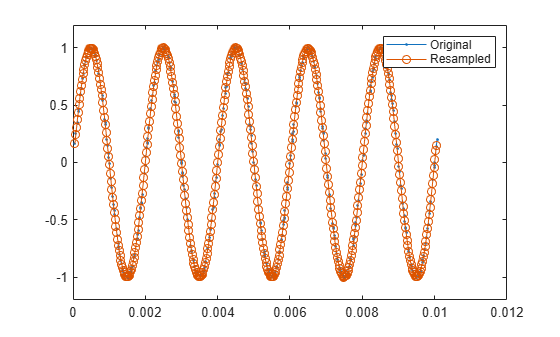### 选择插值方法

`resample` 中的转换算法在输入采样尽可能接近等间距时效果最佳，因此观察采样数据中缺失一部分输入采样时可能发生的情况很有意义。

```irregTx(105:130) = []; x = sin(2*pi*f*irregTx); [y, Ty] = resample(x,irregTx,desiredFs); plot(irregTx,x,'. ') hold on plot(Ty,y,'.-') hold off legend('Original','Resampled') ylim([-1.2 1.2])```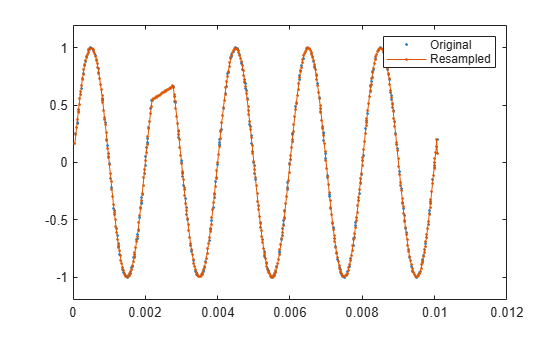```[y, Ty] = resample(x,irregTx,desiredFs,'spline'); plot(irregTx,x,'. ') hold on plot(Ty,y,'.-') hold off legend('Original','Resampled using ''spline''') ylim([-1.2 1.2])```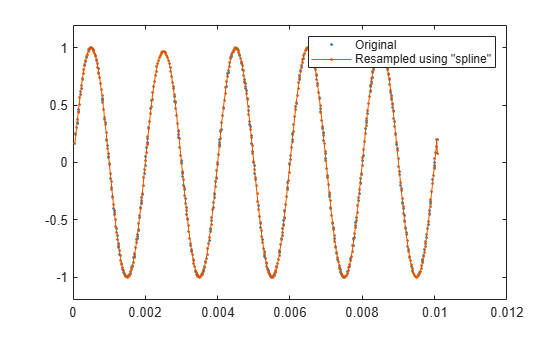### 控制插值网格

```w = 2*pi*3; d = .1002; z = sin(d); a = cos(d); t = [0:0.05:2 3:8]; x = 1 - exp(-z*w*t).*cos(w*a*t-d)/a; plot(t,x,'.-')```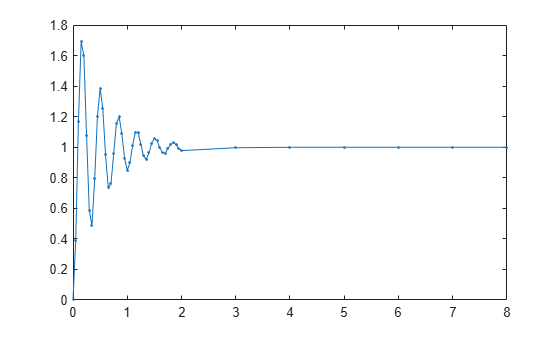```Fs = 100; [y, Ty] = resample(x,t,Fs); plot(t,x,'. ') hold on plot(Ty,y) hold off legend('Original','Resampled (default settings)')````avgFs = (numel(t)-1) /(t(end)-t(1))`
```avgFs = 5.7500 ```

```Fgrid = 7; [p,q] = rat(Fs/Fgrid)```
```p = 100 ```
```q = 7 ```
```[y, Ty] = resample(x,t,Fs,p,q); plot(t,x,'.') hold on plot(Ty,y) hold off legend('Original','Resampled (custom P and Q)')```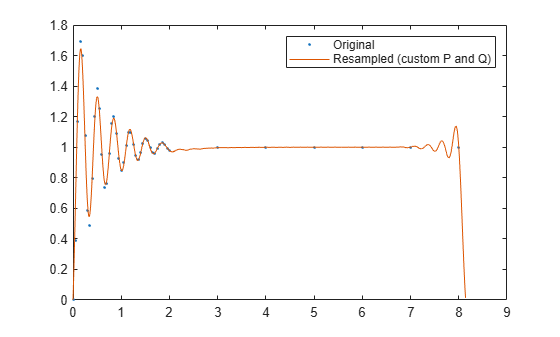### 指定抗混叠滤波器

```load engineRPM plot(t,x,'.') xlabel('Time (s)') ylabel('RPM')```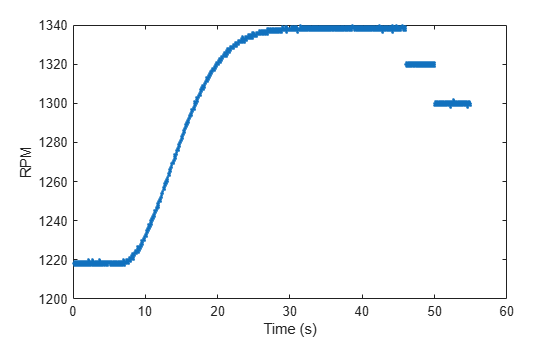```plot(t,x,'.') xlim([20 23])```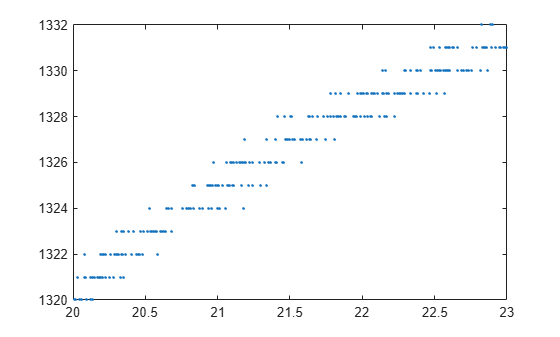```desiredFs = 10; [y,ty] = resample(x,t,desiredFs); plot(t,x,'.') hold on plot(ty,y,'.-') hold off xlabel('Time') ylabel('RPM') legend('Original','Resampled') xlim([20 23])```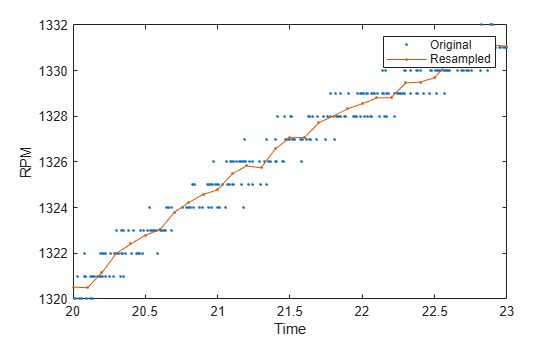`nominalFs = 100;`

```p = 1; q = 10;```

```% ensure an odd length filter n = 10*q+1; % use .25 of Nyquist range of desired sample rate cutoffRatio = .25; % construct lowpass filter lpFilt = p * fir1(n, cutoffRatio * 1/q); % resample and plot the response [y,ty] = resample(x,t,desiredFs,p,q,lpFilt); plot(t,x,'.') hold on plot(ty,y) hold off xlabel('time') ylabel('RPM') legend('Original','Resampled (custom filter)','Location','best') xlim([20 23])```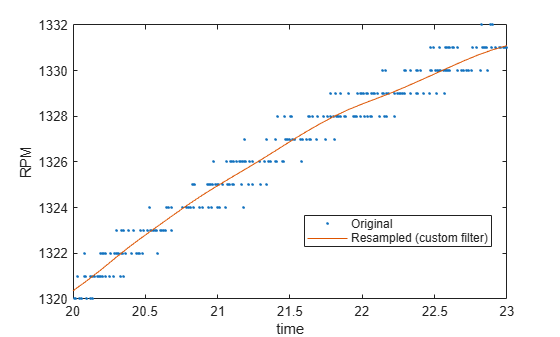### 去除端点效应

```plot(t,x,'.',ty,y) xlabel('time') ylabel('RPM') legend('original','resampled (custom filter)','Location','best')```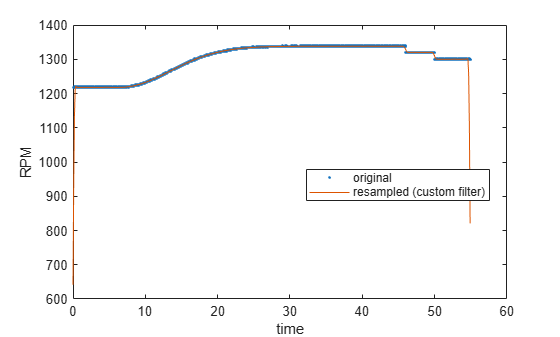```% compute slope and offset (y = a1 x + a2) a(1) = (x(end)-x(1)) / (t(end)-t(1)); a(2) = x(1); % detrend the signal xdetrend = x - polyval(a,t); plot(t,xdetrend)```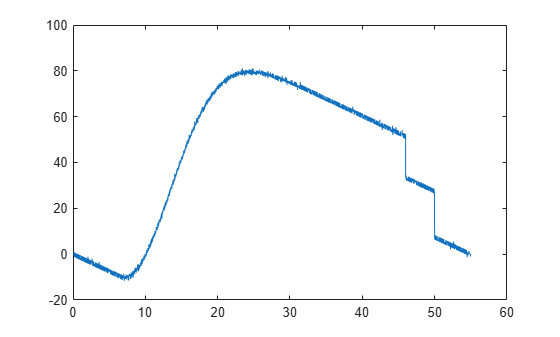```[ydetrend,ty] = resample(xdetrend,t,desiredFs,p,q,lpFilt); y = ydetrend + polyval(a,ty); plot(t,x,'.',ty,y) xlabel('Time') ylabel('RPM') legend('Original','Resampled (detrended, custom filter)','Location','best')```